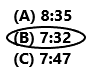# Texas Go Math Grade 2 Lesson 18.3 Answer Key Time to the Minute

Refer to our Texas Go Math Grade 2 Answer Key Pdf to score good marks in the exams. Test yourself by practicing the problems from Texas Go Math Grade 2 Lesson 18.3 Answer Key Time to the Minute.

## Texas Go Math Grade 2 Lesson 18.3 Answer Key Time to the Minute

Problem Solving

Look at the time. Draw the clock hands to show the same time.

Question 7.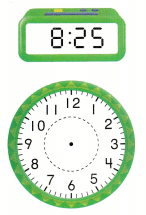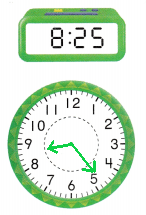The time is 8 : 25.
Explanation:
The time is showing 8 hours and 25 minutes. So, hour hand should be after 8 and near to 9. Minute hand should be pointing to number 5 in the clock.

Question 8.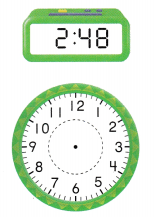The time is 2 : 48.
Explanation:
The time is showing 2 hours and 48 minutes. So, hour hand should be after 2 and near to 3. Minute hand should be between 9 and 10 in the clock.

Question 9.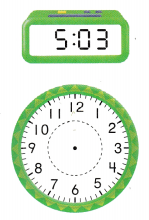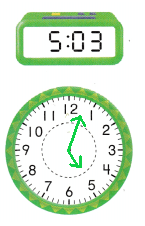The time 5 : 03.
Explanation:
The time is showing 5 hours and 3 minutes. So, hour hand should be at 5. Minute hand should be in between 12 and 1 in the clock.

Question 10.
Multi-Step Look at the clocks to see when Rachel started each activity.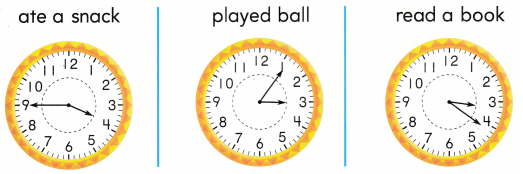Write the times of the activities in order.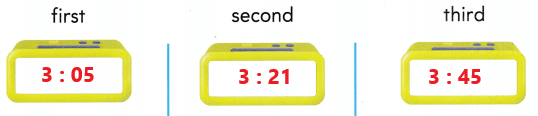Explanation:
Rachel started first played a ball at 3 : 05. Second he read a book at 3 : 21. Third he ate a snack at 3 : 45.

Question 11.
Jamie ate a snack 15 minutes before 4:00.
At what time did Jamie eat a snack?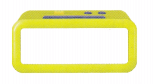Explanation:
Jamie ate a snack 15 minutes before 4:00. Which means Jamie ate a snack at 3 : 45.

Question 12.
Apply This clock shows the time that Miss Grant went to work at the library. Which digital clock shows the same time as the clock to the right?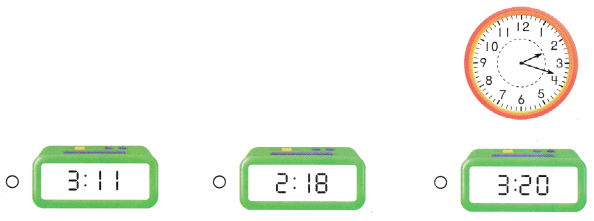Explanation:
The clock shows the time that Miss Grant went to work at the library. The second digital clock shows the same time as the clock to the right. So, put a tick mark to the digital clock with a time 2 : 18.

Question 13.
Representations Kyla started to read at the time shown on this digital clock. Which clock shows the same time?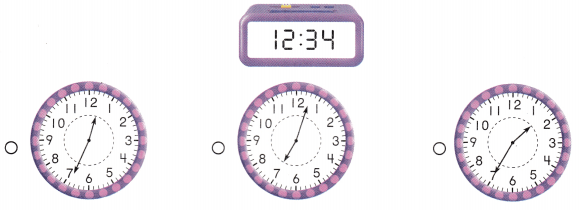Explanation:
Kyla started to read at the time shown on the digital clock. The first clock shows the same time as the digital clock to the right. So, put a tick mark to the digital clock with a time 12 : 34.

Question 14.
Texas Test Prep What time is shown on this clock?
(A) 12:30
(B) 6:03
(C) 6:30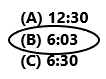Option B is correct.
Explanation:
The time shown on the above clock is 6 : 03. So, draw a circle for option B.

Take Home Activity

• Name a time to the minute. Ask your child to describe where the clock hands point for this time.

### Texas Go Math Grade 2 Lesson 18.3 Homework and Practice Answer Key

Look at the clock hands. Write the time.

Question 1.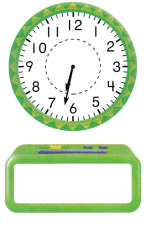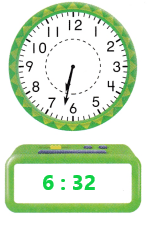The time is 6 : 32.
Explanation:
In the above clock the hour hand is after 6 and near to 7. The minute hand is in between 6 and 7 in the clock. So, the time is 6 : 32 which means 6 hours and 32 minutes.

Question 2.The time is 3 : 18.
Explanation:
In the above clock the hour hand is after 3 and near to 4. The minute hand is in between 3 and 4 in the clock. So, the time is 3 : 18 which means 3 hours and 18 minutes.

Question 3.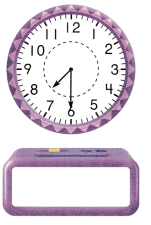The time is 7 : 30.
Explanation:
In the above clock the hour hand is after 7 and near to 8. The minute hand is on the number 6 in the clock. So, the time is 7 : 30 which means 7 hours and 30 minutes.

Problem Solving

Question 4.
Multi-Step Look at the clocks to see when Bonnie started each activity.Write the times of the activities in order. Start with the activity Bonnie did first.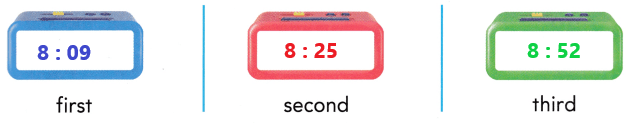Explanation:
Bonnie first wakes up at 8 : 09. Second he gets dressed up at 8 : 25. Third he eats breakfast at 8 : 52.

Texas Test Prep

Question 5.
What time is shown on this clock?
(A) 12:15
(B) 3:01
(C) 3:30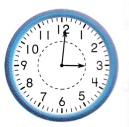Option B is correct.
Explanation:
The time shown on the above clock is 3 : 01. So, draw a circle for option B.

Question 6.
This clock shows the time that Evan watches TV. What time is shown on the clock?(A) 11:28
(B) 4:55
(C) 12:30The time shown on the above clock is 11 : 28.
So, option A is correct.
Explanation:
Evan watches TV at 11: 28. So, draw a circle for option A.

Question 7.
This clock shows the time that Abby calls her friend. What time is shown on the clock?
(A) 3:45
(B) 8:15
(C) 3:42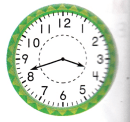The time shown on the above clock is 3 : 42.
Option C is correct.
Explanation:
Abby calls her friend at 3 : 42. So, draw a circle for option C.

Question 8.
What time is shown on this clock?(A) 8:35
(B) 7:32
(C) 7:47